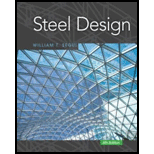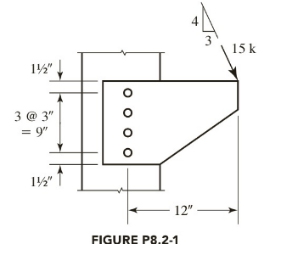Chapter 8, Problem 8.2.1P### Steel Design (Activate Learning wi...

6th Edition
Segui + 1 other
ISBN: 9781337094740

#### Solutions

Chapter
Section### Steel Design (Activate Learning wi...

6th Edition
Segui + 1 other
ISBN: 9781337094740
Textbook Problem

# Use an elastic analysis to determine the maximum bolt shear in the bracket connection of Figure P8.2-1.To determine

The maximum bolt shear force in the given bracket connection by using an elastic analysis.

22.253kips

Explanation

Given:Calculation:

Determine the direct shear components:

Px=35(15)=9kipsPy=45(15)=12kipsPcx=94=2.25kipsPcy=124=3kips

Eccentricity:

ex=12in.ey=9+1.54.5=6in.

M=9(6)+12(12)=198inkips (Clockwise)

(x2+y2)=2[(4.5)2+(1.5)2]=45in.2

Top bolt is critical.

x=0y=92=4.5in.pmx=My(x2+y2)=198(4.5)45=19.8kipspx=2.25+19.8=22.05kipspy=3kipsp=(px)2+(py)2=(22.05)2+(3)2=22.253kips

Conclusion:

The maximum bolt shear force in the given bracket connection is 22.253kips.

#### The Solution to Your Study Problems

Bartleby provides explanations to thousands of textbook problems written by our experts, many with advanced degrees!

Get Started

## Additional Engineering Solutions

#### What is comparison measurement?

Precision Machining Technology (MindTap Course List)

#### What are the three main task patterns? Provide an example of each.

Systems Analysis and Design (Shelly Cashman Series) (MindTap Course List)

#### Determine the resultant of the forces shown.

International Edition---engineering Mechanics: Statics, 4th Edition

Find all the answers to your study problems with bartleby.
Textbook solutions plus Q&A. Get As ASAP arrow_forward